# Algorithm, Pseudocode, Programs, and Flowcharts

## Algorithm, Pseudocode, Programs, and Flowcharts

In this article, I am going to discuss Algorithms, Pseudocode, Programs, and Flowcharts in detail. Please read our previous article where we discussed Programming Methodologies. At the end of this article, you will understand mostly What are Algorithms, what is Pseudocode, What are Programs, and what are Flowcharts.

##### Algorithm, Pseudocode, Programs, and Flowcharts

Algorithm: An algorithm is a step-by-step procedure for solving a computational problem. It is a process or set of rules to be followed in calculations or other problem-solving operations.

Program: A program is a step-by-step machine instruction used for solving any problem or computational task.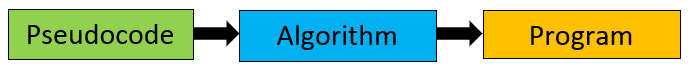##### Difference between Algorithm and Program

Programs have been written recently but Algorithms have appeared for centuries. As a common practice, mathematicians or scientists have been devising procedures for solving computational problems. Those working on problems were giving solutions in form of step-by-step procedures known as algorithms. Now we want that same procedure must be followed by machines so we are writing programs.

An algorithm basically means how to solve a problem. First, we need to learn a few analytical or problem-solving skills to write an algorithm.

###### Example:
1. Let’s consider a chef who knows how to prepare a dish then he/she can easily prepare the recipe of that dish.
2. Let’s consider a chemist who is well versed with different chemical reactions then he/she can easily prepare a chemical formula applying those reactions.

Once an algorithm is prepared, we need to convert it into a Program so that the computer can execute it and perform the computational task. Any programming language can be used to write a program but it must strictly follow the syntax of that programming language.

##### What is Pseudocode?

Pseudocode is an artificial and informal language that helps programmers in developing algorithms. It is basically a “text-based” detail (algorithmic) design tool.

##### Algorithm and Program Example:

So here I have an example algorithm as well as a C++ program that is not a complete program is just a function.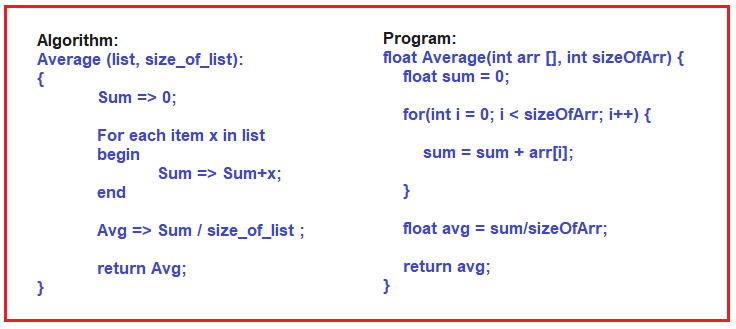##### Let us Understand the Algorithm.

The Algorithm is for finding the average of the list of elements. That is, we have a collection of elements and we want to find out the average. First, we assign 0 to Sum. Then for each element x in the list, we begin sum assigned sum+ x i.e. adding each value of x into the sum variable. Then after that, the average is assigned sum by the number of elements, and then, return the average. So, if you read the above algorithm, you can understand how to find the average of a list of elements. Add all of them and divide by the number of elements. That’s it. This is how we write our algorithm using pseudocode.

##### Let us Understand the Program.

Now the same thing for finding the average list of elements, we have written the program using C++ language. It’s a function, it’s not a complete program, just a function inside a program. If we don’t use a semicolon to end the statement, it’s an error, and instead of assignment if we write less than or a hyphen symbol, then also it is an error. So, if you want to store the value then you must use an equal symbol and that is called an assignment.

So, it means you should follow the proper syntax of a language. Because this is not for you. You are writing the program for the compiler to understand and convert it into machine code. You will write a C++ program and that gets converted into machine code or machine language. So, you are actually talking to the compiler. You should talk in such a way that you can easily understand.

If the compiler is not understanding your program, then the compiler cannot convert your program into machine code. So, you should follow the syntax perfectly. That is the reason you have to put some little extra effort into learning programming.

##### What is a Flowchart?

A flowchart is used for showing the flow of control in a program and the sequence of steps involved in a hierarchical manner. It is basically a diagrammatic representation of an algorithm, workflow, or process.

So, if a program is very big then it is very difficult to figure out how the flow of the program is, Flow charts are useful for understanding the program, instead of one is reading the program and understanding it, he can see the flow chart and understand how the program is working.

It is just like if you talk about electrical wiring in a home. Then from where the wires or the cables are moving through the walls. If you have a plan then you can know where exactly they are flowing and where the important points are, everything you can know. Otherwise, if there is any problem with the wiring, then you have to dig the whole wall to find out the problem. If there is a proper plan then you can understand. So before laying the wire or pulling the wires we will make a plan. In the same way, before writing the program we make a flowchart. So based on the flow chart we will write the program. This will help us to understand the program.

##### Use of Flowchart

Flowcharts were highly used at the times of Monolithic Programming. Then later on when the concept of Procedural Programming came into practice, the usage of flowcharts was a little reduced.

##### Steps in the flowchart:

Usually, when we are using a flow chart for the program, it consists of three steps:

1. Input
2. Process
3. Output

We will call it like this. First, it takes some input. Then it will process. Then it will give the output. So, any procedure you take will have similar steps. For example, preparing a dish. Input is the ingredients. That process is the process of making a dish and the output is the dish ready. If you take a chemistry experiment that is done usually in laboratories will have input means chemicals and the vessels or instruments whatever you need. Then the process of what you will do with that and then it gets done successfully. So, every procedure will have these 3 things and the program is also used to look like this.

##### Elements of Flowchart:

Now let us look at the elements of the flow chart. The following image shows the different elements of a flowchart.

Terminal: The oval symbol indicates Start, Stop and Halt in a program’s logic flow. A pause/halt is generally used in programming logic under some error conditions. The terminal is the first and last symbol in the flowchart.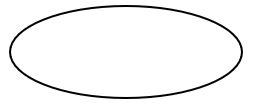Input/Output: A parallelogram denotes any function of input/output type. Program instructions that take input from input devices and display output on output devices are indicated with a parallelogram in a flowchart.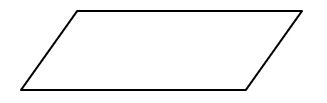Processing: A box represents arithmetic instructions. All arithmetic processes such as addition, subtraction, multiplication, and division are indicated by the action/process symbol.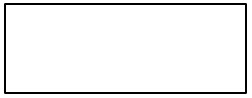Decision: Diamond-shaped symbol represents a decision point. Decision-based operations such as Yes/No, question, or True/False are indicated by diamond shape in the flowchart.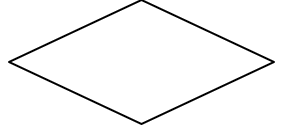Flow lines: Flow lines indicate the exact sequence in which instructions are executed. Arrows represent the direction of flow of control and the relationship among different symbols of the flowchart.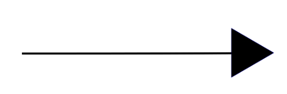Now let us draw a few flow charts and try to understand idea of how flowcharts are used and how they are useful for writing the programs.

##### Flowchart for adding two numbers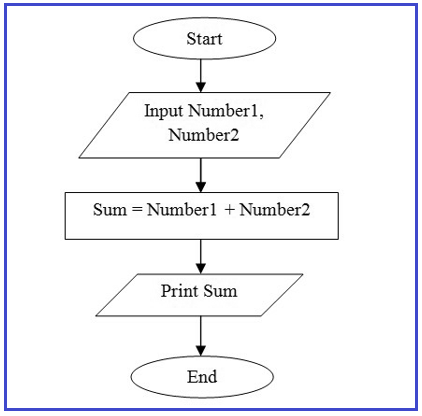Step 1: Start.

Step 2: Declare variables Number1 and Number2.

Step 3: Read values Number1 and Number2.

Step 4: Add Number1 and Number2 and store the result in Sum. (Sum = Number1 + Number2).

Step 5: Display Sum.

Step 6: Stop.

In the next article, I am going to discuss Introduction to .NET Framework. Here, in this article, I try to explain the Algorithm, Pseudocode, Programs, and Flowcharts in detail and I hope you enjoy this Algorithm, Pseudocode, Programs, and Flowcharts article.